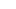Hiak Education Services Pte Ltd

#11-04 Singapore 449408# #25. Question of the Week

May 5, 2019

In the rectangle shown below, the ratio of SA : AB : BQ = 3 : 2 : 5, CR is 3⁄4 of QC and PB = QB. QS is a straight line. Find the ratio of the shaded region to the area the rectangle PQRS.

Solution.

Let area of △SQR = 7 units

Area of △SCQ = 4 units

Area of △PSQ = 7 units

Area of △PAB = 2⁄10 x 7 units = 1.4 units

Shaded region = 4 units + 1.4 units = 5.4 units

Area of PQRS = 7 units + 7 units = 14 units

5.4 : 14

54 : 140

27 : 70

###### Featured Posts

Factors and Multiples

March 17, 20161/1